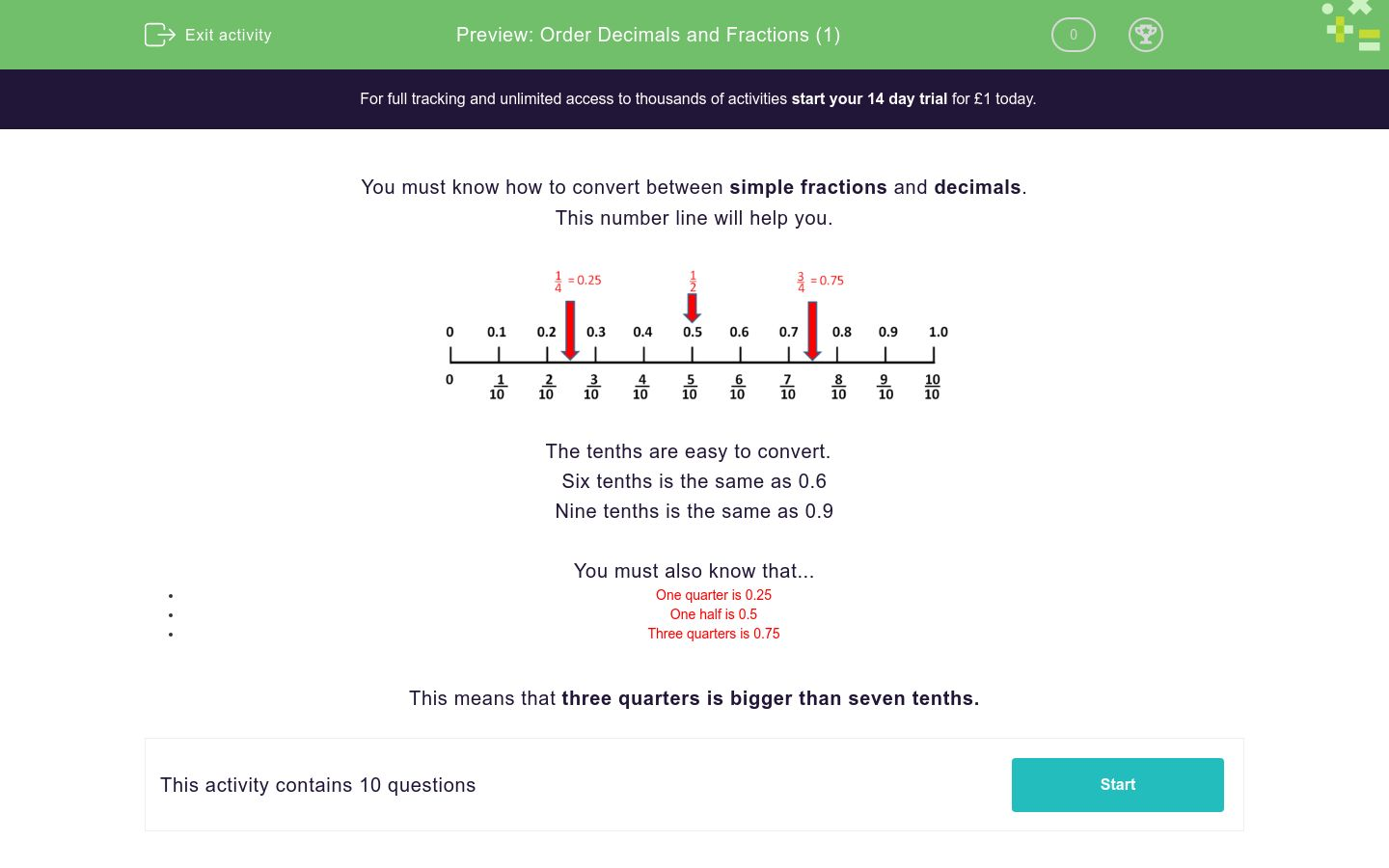# Order Decimals and Fractions (1)

In this worksheet, students use a number line to place simple fractions and decimals in order of size.Key stage:  KS 2

Curriculum topic:  Number: Fractions and Decimals

Curriculum subtopic:  Recognise Decimal Equivalents

Difficulty level:### QUESTION 1 of 10

You must know how to convert between simple fractions and decimals.The tenths are easy to convert.

Six tenths is the same as 0.6

Nine tenths is the same as 0.9

You must also know that...

• One quarter is 0.25
• One half is 0.5
• Three quarters is 0.75

This means that three quarters is bigger than seven tenths.

Put these fractions and decimals in increasing order of size (labelled 1, 2, 3).

 Three tenths 0.4 A quarter

Use the number line to help you.## Column B

1
0.4
2
Three tenths
3
A quarter

Put these fractions and decimals in increasing order of size (labelled 1, 2, 3).

 Five tenths 0.4 0.3

Use the number line to help you.## Column B

1
0.3
2
0.4
3
Five tenths

Put these fractions and decimals in increasing order of size (labelled 1, 2, 3).

 Five tenths A quarter 0.4

Use the number line to help you.## Column B

1
0.4
2
Five tenths
3
A quarter

Put these fractions and decimals in increasing order of size (labelled 1, 2, 3).

 A half 0.4 A quarter

Use the number line to help you.## Column B

1
A half
2
0.4
3
A quarter

Put these fractions and decimals in increasing order of size (labelled 1, 2, 3).

 A half 4 tenths 0.6

Use the number line to help you.## Column B

1
A half
2
0.6
3
4 tenths

Put these fractions and decimals in increasing order of size (labelled 1, 2, 3, 4).

 A half 4 tenths 0.6 0.3

Use the number line to help you.## Column B

1
0.3
2
A half
3
0.6
4
4 tenths

Put these fractions and decimals in increasing order of size (labelled 1, 2, 3, 4).

 A quarter 2 tenths 0.4 0.3

Use the number line to help you.## Column B

1
2 tenths
2
A quarter
3
0.4
4
0.3

Put these fractions and decimals in increasing order of size (labelled 1, 2, 3, 4).

 Three quarters 2 tenths 1 0.1

Use the number line to help you.## Column B

1
2 tenths
2
0.1
3
1
4
Three quarters

Put these fractions and decimals in increasing order of size (labelled 1, 2, 3, 4).

 Three quarters 7 tenths 0.8 A half

Use the number line to help you.## Column B

1
0.8
2
A half
3
Three quarters
4
7 tenths

Put these fractions and decimals in increasing order of size (labelled 1, 2, 3, 4).

 Three quarters 7 tenths 7 0.8

Use the number line to help you.## Column B

1
7 tenths
2
0.8
3
Three quarters
4
7
• Question 1

Put these fractions and decimals in increasing order of size (labelled 1, 2, 3).

 Three tenths 0.4 A quarter

Use the number line to help you.## Column B

1
A quarter
2
Three tenths
3
0.4
• Question 2

Put these fractions and decimals in increasing order of size (labelled 1, 2, 3).

 Five tenths 0.4 0.3

Use the number line to help you.## Column B

1
0.3
2
0.4
3
Five tenths
• Question 3

Put these fractions and decimals in increasing order of size (labelled 1, 2, 3).

 Five tenths A quarter 0.4

Use the number line to help you.## Column B

1
A quarter
2
0.4
3
Five tenths
• Question 4

Put these fractions and decimals in increasing order of size (labelled 1, 2, 3).

 A half 0.4 A quarter

Use the number line to help you.## Column B

1
A quarter
2
0.4
3
A half
• Question 5

Put these fractions and decimals in increasing order of size (labelled 1, 2, 3).

 A half 4 tenths 0.6

Use the number line to help you.## Column B

1
4 tenths
2
A half
3
0.6
• Question 6

Put these fractions and decimals in increasing order of size (labelled 1, 2, 3, 4).

 A half 4 tenths 0.6 0.3

Use the number line to help you.## Column B

1
0.3
2
4 tenths
3
A half
4
0.6
• Question 7

Put these fractions and decimals in increasing order of size (labelled 1, 2, 3, 4).

 A quarter 2 tenths 0.4 0.3

Use the number line to help you.## Column B

1
2 tenths
2
A quarter
3
0.3
4
0.4
• Question 8

Put these fractions and decimals in increasing order of size (labelled 1, 2, 3, 4).

 Three quarters 2 tenths 1 0.1

Use the number line to help you.## Column B

1
0.1
2
2 tenths
3
Three quarters
4
1
• Question 9

Put these fractions and decimals in increasing order of size (labelled 1, 2, 3, 4).

 Three quarters 7 tenths 0.8 A half

Use the number line to help you.## Column B

1
A half
2
7 tenths
3
Three quarters
4
0.8
• Question 10

Put these fractions and decimals in increasing order of size (labelled 1, 2, 3, 4).

 Three quarters 7 tenths 7 0.8

Use the number line to help you.## Column B

1
7 tenths
2
Three quarters
3
0.8
4
7
EDDIE SAYS
7 is 7 whole units
---- OR ----

Sign up for a £1 trial so you can track and measure your child's progress on this activity.

### What is EdPlace?

We're your National Curriculum aligned online education content provider helping each child succeed in English, maths and science from year 1 to GCSE. With an EdPlace account you’ll be able to track and measure progress, helping each child achieve their best. We build confidence and attainment by personalising each child’s learning at a level that suits them.

Get started#### Similar activities you may like

Start your £1 trial today.
Subscribe from £10/month.